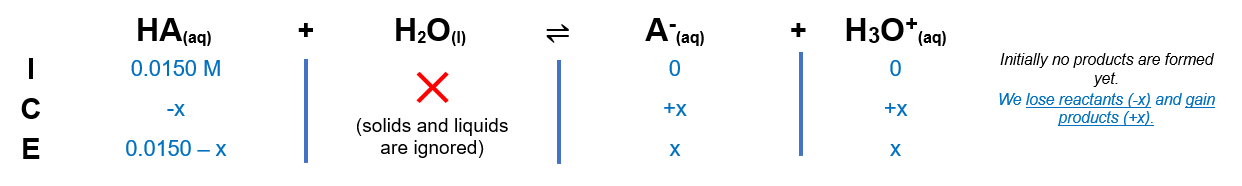# Problem: If enough of a monoprotic acid is dissolved in water to produce a 0.0150 M solution with a pH of 6.05, what is the equilibrium constant for the acid?

###### FREE Expert Solution

The dissociation of HA is as follows:

HA(aq) + H2O(l)  H3O+(aq) + A(aq)

From this, we can construct an ICE table. Remember that liquids are ignored in the ICE table and Ka expression.The Ka expression for HA is:

$\overline{){{\mathbf{K}}}_{{\mathbf{a}}}{\mathbf{=}}\frac{\mathbf{products}}{\mathbf{reactants}}{\mathbf{=}}\frac{\mathbf{\left[}{\mathbf{H}}_{\mathbf{3}}{\mathbf{O}}^{\mathbf{+}}\mathbf{\right]}\mathbf{\left[}{\mathbf{A}}^{\mathbf{-}}\mathbf{\right]}}{\mathbf{\left[}\mathbf{HA}\mathbf{\right]}}}$

85% (278 ratings)###### Problem Details

If enough of a monoprotic acid is dissolved in water to produce a 0.0150 M solution with a pH of 6.05, what is the equilibrium constant for the acid?